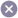Home > Running Total ( Math Logic )

# Running Total ( Math Logic )

Creating a Running Total / Math Logic

Running Total in ARForms provides math logic or in simple language, you can say that when we need calculation of two or more field then this can be achieved by using Running Total.

Running Total is performed in HTML field inside field-options, steps to perform Running Total are as shown below.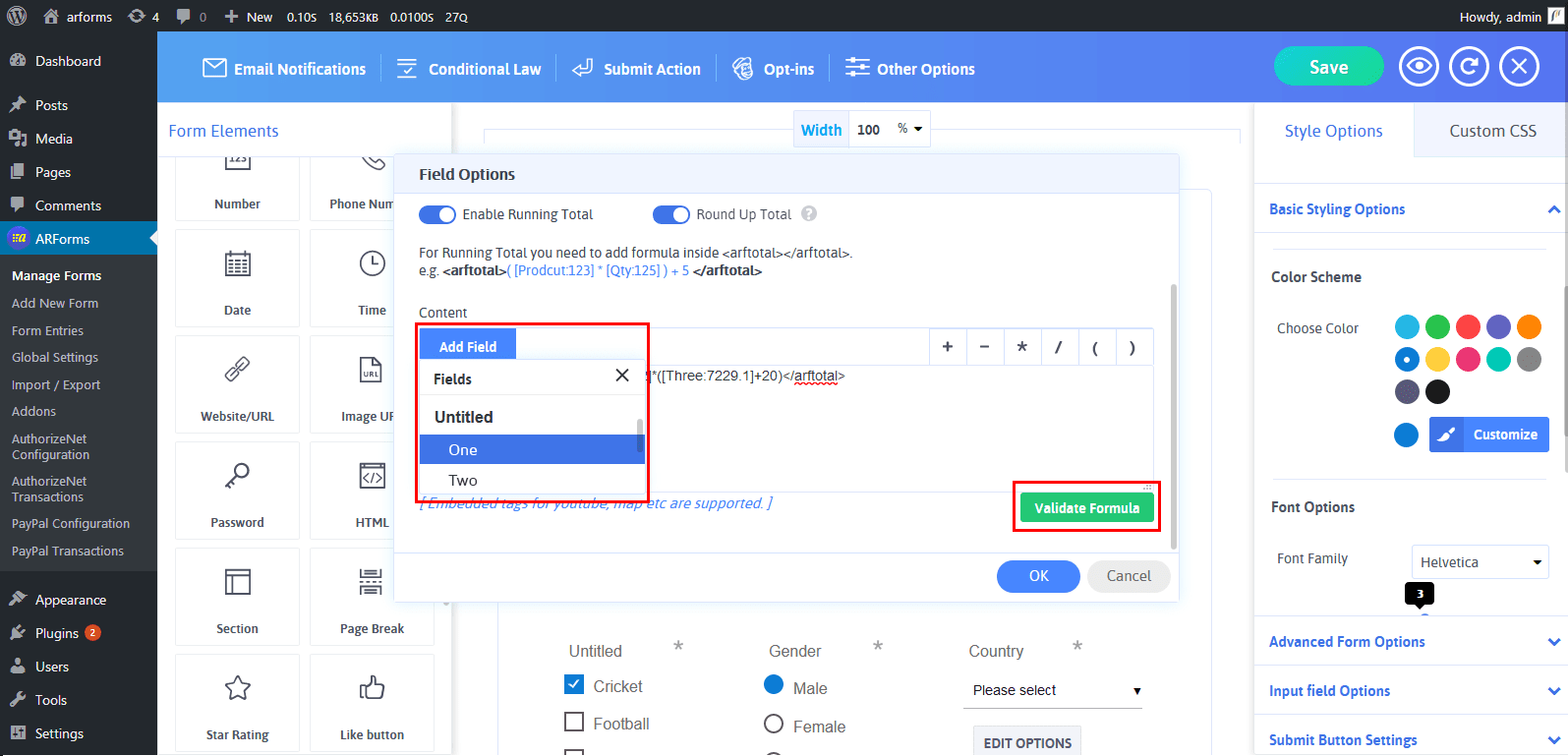When you want to add formulas for math logic, you need to check this checkbox (Enable Running Total). When you will check this checkbox, it will automatically add a pair of tag <arftotal></arftotal> (Opening and closing tag).

You will be able to see a sample formula as displayed. Here, you will be able to see ‘Add Field’ dropdown from where you can add form fields for calculation and list of an operand to do a calculation. Even there is a facility to check formula whether it is valid or not just by clicking on Validate Formula.

Sigma Icon

After adding required running total you will find that a sigma icon will be enabled in HTML field, this “Sigma Icon” specifies that HTML field has “Running Total Enabled”.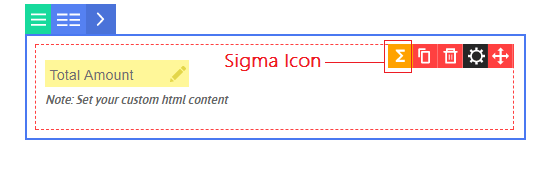Displaying the Calculation in Your Form

Displaying a calculation in your form is very easy,  with ARForms, it will be displayed where you have added an HTML field in which you wrote whole formula or math logic and you can add prefix as per your need before <arftotal></arftotal> tags.

let’s illustrate a case in which we add running total to field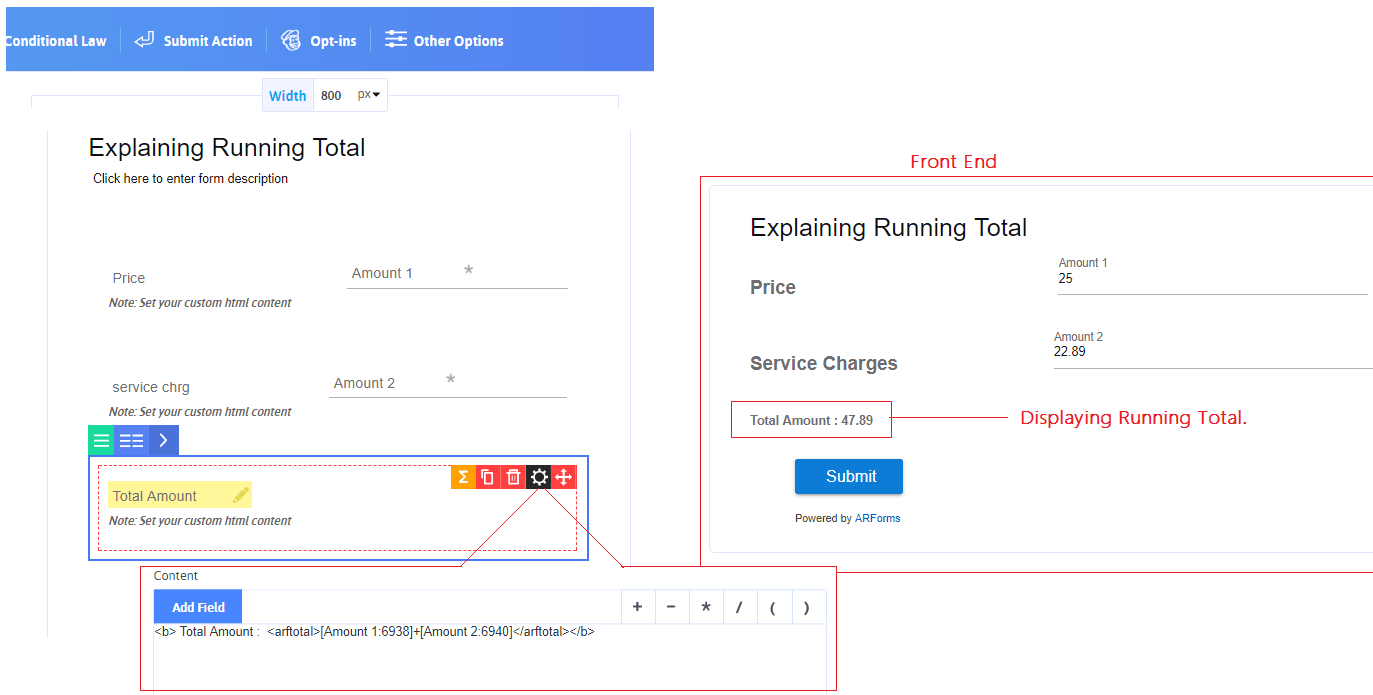Enable Running Total:

When you want to add formulas for math logic, you have to check Enable Running Total checkbox. When you check this checkbox, it will automatically add a pair of`<arftotal>` `</arftotal>` tag. ( Opening and closing tag. ) where your cursor is pointing.

After checked the Enable Running Total, Please confirm that your cursor is pointing between the <arftotal> tag. You need to add formula between opening and closing tag.

After enabling the running total, you will get Form fields to add in formula along with math operators for calculation.

This is the list of all fields which you have already added in editor for your form. Select any field which you want to use for calculation. By selecting field, it will be added to your formula which you can see in HTML content area. Here some inputs will not included such as Range slider, Page Break, Captcha.

Math Operators:

`{ + } Sign:`

It will perform addition operation between fields(operands). Just simply click on button and it will be added to your formula where your cursor is pointing.

`{ - } Sign:`

It will perform subtraction operation between fields(operands). Just simply click on button and it will be added to your formula where your cursor is pointing.

`{ *} Sign:`

It will perform multiplication operation between fields(operands). Just simply click on button and it will be added to your formula where your cursor is pointing.

`{ /} Sign:`

It will perform division operation between fields(operands). Just simply click on button and it will be added to your formula where your cursor is pointing.

`{ ( ) } Brackets:`

Starting and closing bracket used to differentiates and give high priority to particular operation between formulas.

##### example
``([Product:123]*[Qty:125]) + 5``

Validate Formula:

After adding the required fields and operators to the formula, you may want to check that this formula is valid or not. So, this button will check whether your formula is valid or not. If your formula contains any incorrect expression, then it will show you error message.

Round Up Total

Enable this option if you want to round up the total value of the calculation.

For example, if the total is 12.49 then it will round up with 12 and if the total is 12.50 then it will round up with 13.

Running Total widely used to add final amount (result of running total) as a Price in Payment Addon, Lets consider the above illustrated form where `Total Amount` contains sum of two fields ‘price’ & ‘charges’ which is considered as final amount to be paid by user.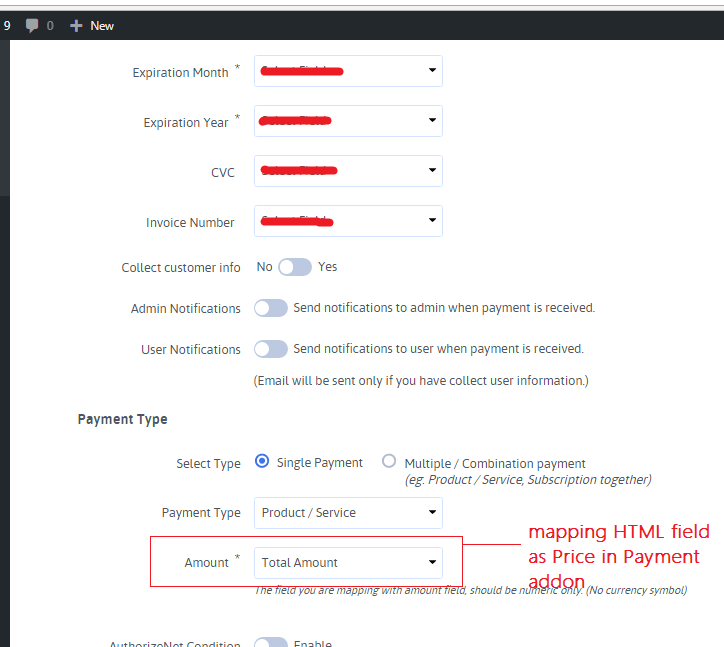## Subscribe To Our Newsletter ForBest Monthly Offers & Discounts!

Be a part of our subscription and stay updated with the latest deals we offer.
Subscribe Me!
No Thanks!## Limited Time Offer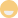We are celebrating 9000 sales